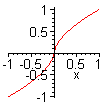Function: &^ - "elementary" fractional powers

Calling sequence:

x &^ p;

Parameters:

x, p - any algebraic expressions. Numerical values of p should be rational numbers.

Description:

Maple's mathematics is largely based on complex numbers rather than real numbers. It uses the "principal branch" of fractional powers. In particular, if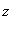is a negative real number and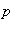is real but not an integer,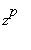is not real. That is not the branch that is generally used in more elementary mathematics, where, for example, the cube root of a negative number would be taken to be negative. The surd function can be used to obtain these "elementary" roots, but it is comparatively clumsy notation and does not cover fractional exponents with numerators other than 1. The &^ operator is intended to address these shortcomings.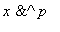is always a branch of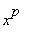.

For positive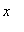,is the same as. For negative, if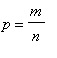is a fraction we have the following cases:

-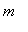and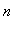both odd: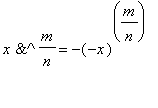-even,odd: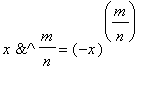-odd,even: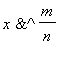is complex.

Whenis not known to be real, andis a fraction,is written as a surd.

Whenis given in decimal form, it is converted to a fraction.

This operator is part of the Maple Advisor Database, and must be read in before use with readlib(`&^`); (note the back quotes).

Examples: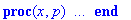> evalc((-1)^(1/3)), surd(-1,3), (-1)&^(1/3);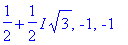> evalc((-1)^(1/4)),
evalc(surd(-1,4)), evalc((-1)&^(1/4));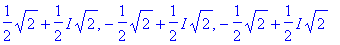> evalc((-1)^(2/3)), (-1) &^(2/3);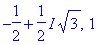> evalc((-1)^(5/8)),evalc((-1)&^(5/8));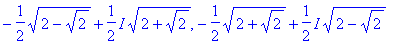> assume(n<0): n &^(3/5), n &^ (4/5);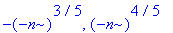> plot(x &^ (2/3), x = -1 .. 1);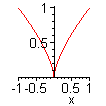> plot(x &^ (3/5), x = -1 .. 1);